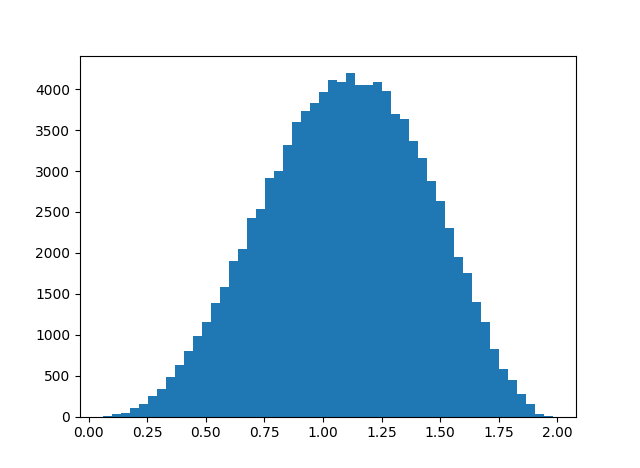# How close is octonion multiplication to being associative?

Quaternion multiplication is associative but not commutative. An earlier post looked at the average size of the commutator xy – yx as a measure of how far quaternion multiplication is from being commutative.

This post looks at an analogous question for octonions. Octonion multiplication is neither commutative nor associative. So in this post we look at the associator of three octonions (xy)z – x(yz) as a measure of how far octonion multiplication is from being associative.

(A post from yesterday looked at how close octonion multiplication comes to being associative in an algebraic sense, looking at weak forms of associativity. This post looks at how close multiplication comes to being associative in an analytical sense, looking at norm distances rather than algebraic identities.)

We will use a simulation to look at the average norm of the octonion associator over the octionions of unit length, analogous to the earlier post that looked at the commutator of the quaternions. We developed code for octonion multiplication in the previous post and will reuse that code here. We also developed code for generating random unit-length octonions in the same post.

Here’s code to find the average norm of the associator and plot a histogram of its values.

```
import matplotlib.pyplot as plt

# omult is octonion multiplication.
# See previous post.
def associator(x, y, z):
return omult(omult(x, y), z) -
omult(x, omult(y, z))

N = 100000
s = 0
h = np.zeros(N)
for n in range(N):
x = random_unit_octonion()
y = random_unit_octonion()
z = random_unit_octonion()
t = norm(associator(x, y, z))
s += t
h[n] = t

print(s/N)
plt.hist(h, bins=50)
plt.show()
```

This gives an average of 1.095 and the histogram below.Update: Greg Egan calculated the exact mean value for the norm of the associator to be 147456/(42875 π) ≈ 1.0947336. Here are the details.

## 2 thoughts on “How close is octonion multiplication to being associative?”

1. Great post!
The function is defined as assoc but used as associator.

2. Thanks.

My code originally used `assoc`, then I decided after I pasted the code into the blog that `associator` would be more readable. I should learn to never edit code in the blog editor!